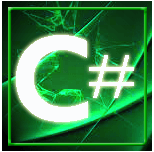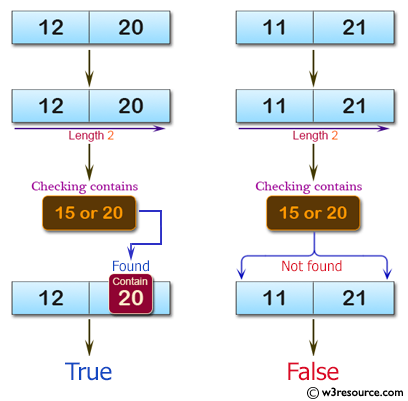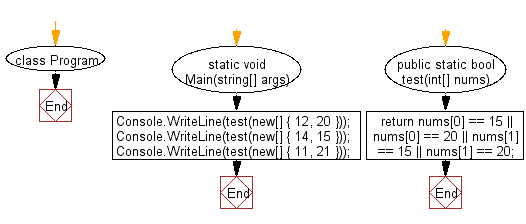﻿ C# Sharp exercises: Check if a given array of integers and length 2, contains 15 or 20. - w3resource# C# Sharp Basic Algorithm Exercises: Check if a given array of integers and length 2, contains 15 or 20

## C# Sharp Basic Algorithm: Exercise-97 with Solution

Write a C# Sharp program to check if a given array of integers and length 2, contains 15 or 20.

Pictorial Presentation:Sample Solution:-

C# Sharp Code:

``````using System;
namespace exercises
{
class Program
{
static void Main(string[] args)
{
Console.WriteLine(test(new[] { 12, 20 }));
Console.WriteLine(test(new[] { 14, 15 }));
Console.WriteLine(test(new[] { 11, 21 }));
}
public static bool test(int[] nums)
{
return nums == 15 || nums == 20 || nums == 15 || nums == 20;
}
}
}
```
```

Sample Output:

```True
True
False```

Flowchart:C# Sharp Code Editor:

Improve this sample solution and post your code through Disqus

What is the difficulty level of this exercise?

﻿

New Content: Composer: Dependency manager for PHP, R Programming